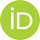Title: Algorithmic properties of equivalence relations Language: English Authors: Fokina, EkaterinaQualification level: Doctoral Keywords: logic; algorithmic Advisor: Baaz, Matthias Issue Date: 2016 Number of Pages: 61 Qualification level: Doctoral Abstract: Equivalence relations formalize the idea of resemblance between mathematical objects. In the context of computable structure theory, equivalence relations are a useful tool to study the effectiveness of mathematical structures. On the other hand, the study of algorithmic properties of equivalence relations themselves is an active area of investigation with many open questions attracting a lot of attention of specialists in the field. We only consider countable structures in computable languages. We identify structures with their atomic diagrams.In particular, a structure is computable if its atomic diagram is a computable subset of the natural numbers. We consider the above mentioned directions to study the role of equivalence relations in computable structure theory. We start by looking at isomorphisms of bounded Turing degree. For a degree d, we say that a computable structure A is d-categorical if for every its isomorphic computable copy B there exists a d-computable isomorphims between A and B. First we concider the connections between algebraic, descriptive and algorithmic properties of mathematical structure through the notions of 0n categoricity and relative 0n categoricity. We look at natural classes of structures including various kinds of groups, Boolean algebras, Fraissé limits, etc. The results form a part of the paper "Computability-Theoretic Categoricity and Scott Families" by E. Fokina, V. Harizanov and D. Turetsky. We then answer the question how hard it is to describe the property of eective categoricity for structures with various algorithmic restrictions. The main notion here is the notion of index sets. We give the exact estimation of complexity for structures that are n-decidable categorical relative to m-decidable presentations, for various m, n e w. The results appear in the paper -Index sets of n-decidable structures categorical relative to m-decidable presentations-, joint with S. Goncharov, V. Harizanov, O. Kudinov and D. Turetsky. Finally, we employ degrees of atomic diagrams of structures to characterize the inherent complexity of equivalence classes of structures, up to various equivalence relations. Here we introv Summary duce the notion of degree spectra relative to equivalence relations. We study the degree spectra of structures relative to n-equivalence (two structures are n-equivalent if there n-theories coincide). The presented results form a part of the paper "Degree Spectra of Structures relative to Equivalence Relations" by E. Fokina, P. Semukhin and D. Turetsky. For all the new results provided in this thesis, the main contribution to proving and writing them down was done by E. Fokina. Introduction uses material from the paper "Computable Model Theory" by E. Fokina, V. Harizanov and A. Melnikov. URI: https://resolver.obvsg.at/urn:nbn:at:at-ubtuw:1-7962http://hdl.handle.net/20.500.12708/2922 Library ID: AC13361542 Organisation: E104 - Institut für Diskrete Mathematik und Geometrie Publication Type: ThesisHochschulschrift Appears in Collections: Thesis

#### Files in this item:

File Description SizeFormat
Fokina Ekaterina - 2016 - Algorithmic properties of equivalence relations.pdf476.25 kBAdobe PDF#### Page view(s)

12
checked on Apr 1, 2021

#### Download(s)

74
checked on Apr 1, 2021#### Google ScholarTM

Check

Items in reposiTUm are protected by copyright, with all rights reserved, unless otherwise indicated.